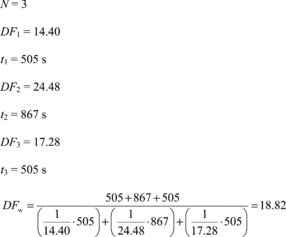# Title 40

## SECTION 1066.610

### 1066.610 Dilution air background correction.

§ 1066.610 Dilution air background correction.

(a) Correct the emissions in a gaseous sample for background using the following equation: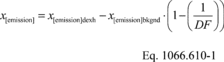Where: x[emission]dexh = measured emission concentration in dilute exhaust (after dry-to-wet correction, if applicable). x[emission]bkgnd = measured emission concentration in the dilution air (after dry-to-wet correction, if applicable). DF = dilution factor, as determined in paragraph (b) of this section.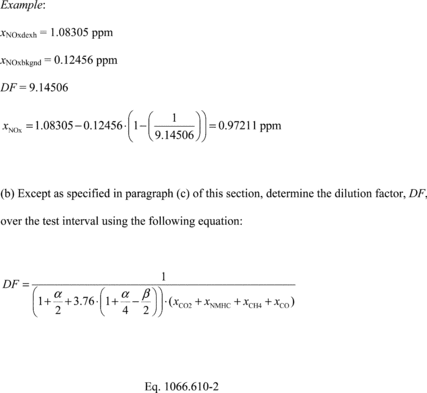Where: xCO2 = amount of CO2 measured in the sample over the test interval. xNMHC = amount of C1-equivalent NMHC measured in the sample over the test interval. xCH4 = amount of CH4 measured in the sample over the test interval. xCO = amount of CO measured in the sample over the test interval. a = atomic hydrogen-to-carbon ratio of the test fuel. You may measure a or use default values from Table 1 of 40 CFR 1065.655. b = atomic oxygen-to-carbon ratio of the test fuel. You may measure b or use default values from Table 1 of 40 CFR 1065.655.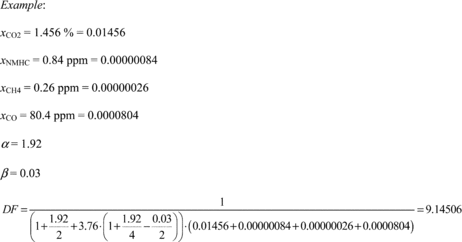(c) Determine the dilution factor, DF, over the test interval for partial-flow dilution sample systems using the following equation: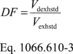Where:

Vdexhstd = total dilute exhaust volume sampled over the test interval, corrected to standard reference conditions.

Vexhstd = total exhaust volume sampled from the vehicle, corrected to standard reference conditions.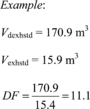(d) Determine the time-weighted dilution factor, DFw, over the duty cycle using the following equation: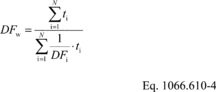Where: N = number of test intervals. i = test interval number t = duration of the test interval. DF = dilution factor over the test interval. Example: